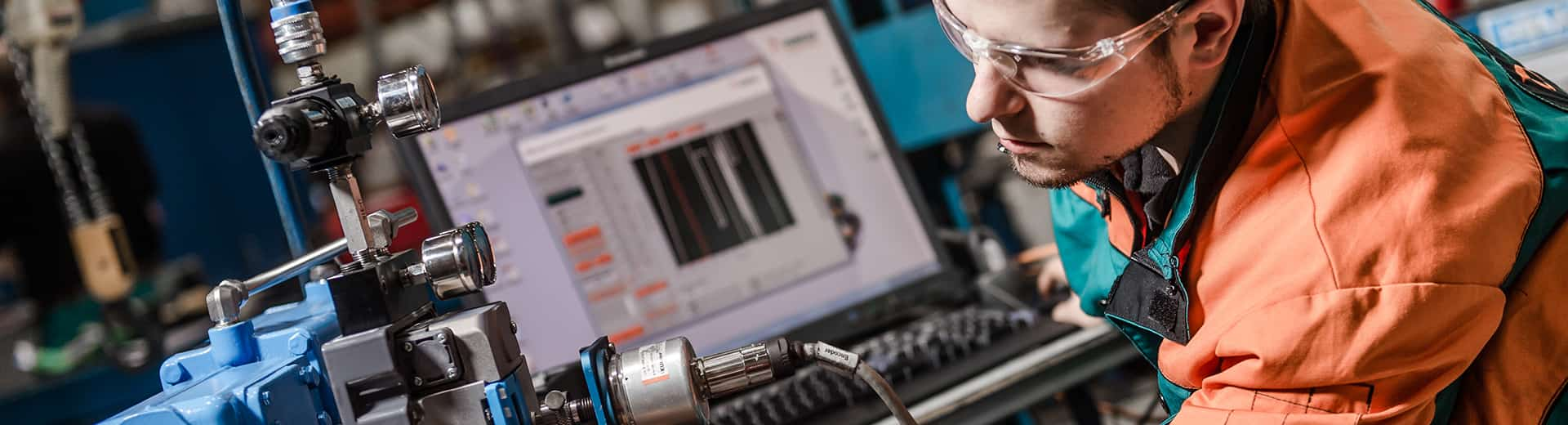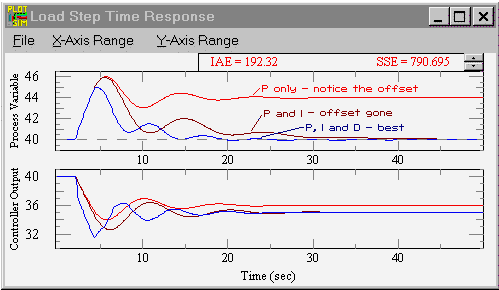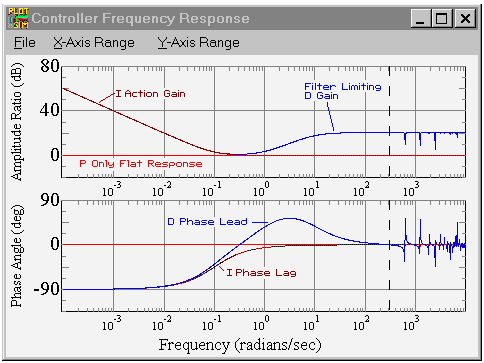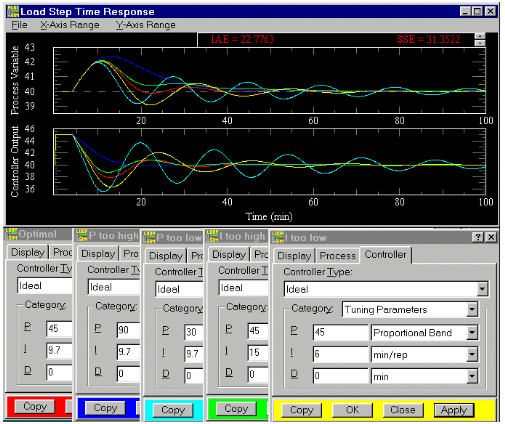# PID tuning tutorial

## What is PID? Learn how PID controls work in real industrial control systems## PID Tuning tutorial overview

PID stands for Proportional, Integral, Derivative. Controllers are designed to eliminate the need for continuous operator attention. Cruise control in a car and a house thermostat are common examples of how controllers are used to automatically adjust some variable to hold the measurement (or process variable) at the set-point. The set-point is where you would like the measurement to be. Error is defined as the difference between set-point and measurement.

(error) = (set-point) - (measurement) The variable being adjusted is called the manipulated variable which usually is equal to the output of the controller. The output of PID controllers will change in response to a change in measurement or set-point. Manufacturers of PID controllers use different names to identify the three modes. These equations show the relationships:

P = 100/gain

I = 1/reset (units of time)

D = rate = pre-act (units of time)

Depending on the manufacturer, integral or reset action is set in either time/repeat or repeat/time. One is just the reciprocal of the other. Note that manufacturers are not consistent and often use reset in units of time/repeat or integral in units of repeats/time. Derivative and rate are the same.

Choosing the proper values for P, I, and D is called "PID Tuning".

## Proportional band

With proportional band, the controller output is proportional to the error or a change in measurement (depending on the controller).

CONTROLLER OUTPUT = (ERROR)*100/(PROPORTIONAL BAND)

With a proportional controller offset (deviation from set-point) is present. Increasing the controller gain will make the loop go unstable. Integral action was included in controllers to eliminate this offset.

## Integral

With integral action, the controller output is proportional to the amount of time the error is present. Integral action eliminates offset.

CONTROLLER OUTPUT = (1/INTEGRAL) (Integral of) e(t) d(t)Notice that the offset (deviation from set-point) in the time response plots is now gone. Integral action has eliminated the offset. The response is somewhat oscillatory and can be stabilized some by adding derivative action. (Graphic courtesy of Expertune PID Loop Optimitizer)

Integral action gives the controller a large gain at low frequencies that results in eliminating offset and "beating down" load disturbances. The controller phase starts out at –90 degrees and increases to near 0 degrees at the break frequency. This additional phase lag is what you give up by adding integral action. Derivative action adds phase lead and is used to compensate for the lag introduced by integral action.

## Derivative

With derivative action, the controller output is proportional to the rate of change of the measurement or error. The controller output is calculated by the rate of change of the measurement with time.

CONTROLLER OUTPUT = DERIVATIVE (dm)/(dt)

Where m is the measurement at time t.

Some manufacturers use the term rate or pre-act instead of derivative. Derivative, rate, and pre-act are the same thing.

DERIVATIVE = RATE = PRE ACT

Derivative action can compensate for a changing measurement. Thus derivative takes action to inhibit more rapid changes of the measurement than proportional action. When a load or set-point change occurs, the derivative action causes the controller gain to move the "wrong" way when the measurement gets near the set-point. Derivative is often used to avoid overshoot.

Derivative action can stabilize loops since it adds phase lead. Generally, if you use derivative action, more controller gain and reset can be used.With a PID controller the amplitude ratio now has a dip near the center of the frequency response. Integral action gives the controller high gain at low frequencies, and derivative action causes the gain to start rising after the "dip". At higher frequencies the filter on derivative action limits the derivative action. At very high frequencies (above 314 radians/time; the Nyquist frequency) the controller phase and amplitude ratio increase and decrease quite a bit because of discrete sampling. If the controller had no filter the controller amplitude ratio would steadily increase at high frequencies up to the Nyquist frequency (1/2 the sampling frequency). The controller phase now has a hump due to the derivative lead action and filtering. (Graphic courtesy of ExperTune Loop Simulator.)

The time response is less oscillatory than with the PI controller. Derivative action has helped stabilize the loop.

## Control loop tuning

It is important to keep in mind that understanding the process is fundamental to getting a well designed control loop. Sensors must be in appropriate locations and valves must be sized correctly with appropriate trim.

In general, for the tightest loop control, the dynamic controller gain should be as high as possible without causing the loop to be unstable. Choosing a controller gain is accomplished easily with PID Tuning Software

## Fine tuning "rules"

This picture (from the Loop Simulator) shows the effects of a PI controller with too much or too little P or I action. The process is typical with a dead time of 4 and lag time of 10. Optimal is red.

You can use the picture to recognize the shape of an optimally tuned loop. Also see the response shape of loops with I or P too high or low. To get your process response to compare, put the controller in manual change the output 5 or 10%, then put the controller back in auto.

P is in units of proportional band. I is in units of time/repeat. So increasing P or I, decreases their action in the picture.## Starting PID settings for common control loops

 Loop type PB % Integral min/rep Integral rep/min Derivative min Valve type Flow 50 to 500 0.005 to 0.05 20 to 200 none Linear or modified percentage Liquid pressure 50 to 500 0.005 to 0.05 20 to 200 none Linear or modified percentage Gas pressure 1 to 50 0.1 to 50 0.02 to 10 0.02 to 0.1 Linear Liquid level 1 to 50 1 to 100 0.1 to 1 0.01 to 0.05 Linear or modified percentage Temperature 2 to 100 0.2 to 50 0.02 to 5 0.1 to 20 Equal percentage Chromatograph 100 to 2000 10 to 120 0.008 to 0.1 0.1 to 20 Linear

These settings are rough, assume proper control loop design, ideal or series algorithm and do not apply to all controllers. Use Expertune's PID Loop Optimizer to find the proper PID settings for your process and controller. (From Process Control Systems (Shinskey) p.99 and Tuning and Control Loop Performance (McMillan) p 39)